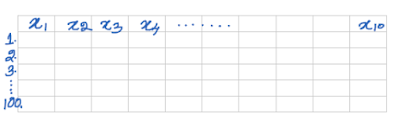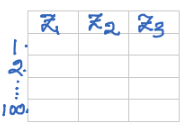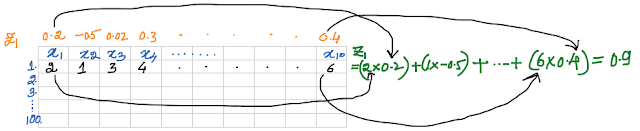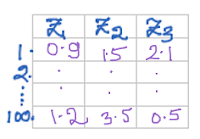# Data Science Simplified

Learning the Machine Learning, in a Human-friendly Way

### Demystifying Principal Component Analysis (PCA): A Beginner's Guide with Intuitive Examples & Illustrations

In this post, let us understand

• What is Principal Component Analysis (PCA)
• When to use it and what are the advantages
• How to perform PCA in Python with an example

### What is Principal Component Analysis (PCA)?

Principal Component Analysis is an unsupervised data analysis technique. It is used for dimensionality reduction. Okay, now what is dimensionality reduction?

In simple terms, dimensionality reduction refers to reducing the number of variables. But if we reduce the number of variables, don’t we lose the information as well?

Yes, we do lose some information. Well if eliminate variables (directly dropping some of the variables), then we may lose significant amount of information. But instead of this, if we create new variables from the existing variables (i.e. feature extraction), then we may not lose much of the information.

In PCA, the objective is to reduce the variables in such a way that we are able to retain as much information as possible. Okay, now how to do it?

#### Simple example to illustrate PCA

Well, imagine we have a dataset which contains data on ten variables (x1 to x10) for 100 observations. The dataset looks something like this:Dataset - ten variables (x1 to x10) and 100 observations

Now, we have to reduce this dataset into three variables without losing much information. It will look something like this:First three principal components

That doesn't mean that there will be only three principal components. In fact, if there are 10 variables, there will be 10 principal components. But if we are going to use all 10 principal components, then what is the use of performing PCA, we could directly use the 10 original variables, isn't it?

#### How many Principal Components should we retain?

If the initial principal components explain maximum information (or variance) present in the data, then it is better.

Let us say if you want at least 80% of the information present in the data to be retained, how many PCs would you need?

If the total variance or the information present in the data is 100% or (or 1), then using the eigenvalues, we can find out how much of the information is explained by each of the PCs.

In the following graph, you can see that first Principal Component (PC) accounts for 70%, second PC accounts for 20% and so on. The variance explained by components decline with each component. If we retail first two PCs, then the cumulative information retained is 70% + 20% = 90% which meets our 80% criterion.PCs and explained variance - Scree plot

#### How Principal Component Scores are calculated?

Principal Component scores are obtained by multiplying PCA loadings with the corresponding x values. PCA loadings are highlighted in yellow. Hence each principal component is a linear combination of the observed variables.Calculating First PC scoresCalculating Second PC scoresPCA scores

Instead of using original data, we can now use PCA scores for our further analysis such as regression or classification model.

#### How Principal Components are generated?

Imagine our dataset contains only two variables and green dots represent observations, then first PC tries to retain as much information as possible.First PC

Second PC will be perpendicular to the first PC and tries to explain maximum remaining information.Second PC

#### What are the advantages of PCA?

• Popular method for dimensionality reduction
• Helps to overcome the problem of multicollinearity
• When there are too many variables and you don't know which ones to drop

• Major limitation is the assumption of linearity.
• And it is useful for quantitative data, not recommended for qualitative data.
• Interpreting PCs is difficult when compared to original variables

#### How to perform PCA in Python with an example

Let us see how to perform PCA in sklearn  using the iris dataset.

Since PCA is affected by the units of features, we have to standardize the features before running PCA.

Number of components can be left blank while running PCA for the first time since we will not be knowing the explained variance by each of the PCs.

In our case, 73% of the information explained by the first PC, while the second PC explains 23% of the information.

#### Conclusion

In this post, we have explored